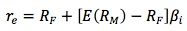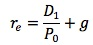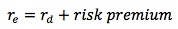Subject 3. Cost of Common Equity
#cfa #cfa-level-1 #corporate-finance #cost-of-capital #has-images
The cost of common equity (re) is the rate of return stockholders require on common equity capital the firm obtains. It has no direct costs but is related to the opportunity cost of capital: if the firm cannot invest newly obtained equity or retained earnings and earn at least re, it should pay these funds to its stockholders and let them invest directly in other assets that do provide this return. Firms should earn on retained earnings at least the rate of return shareholders expect to earn on alternative investments with equivalent risk.

Estimating the cost of common equity is challenging due to the uncertain nature of the amount and timing of future cash flows.

The CAPM Approachwhere RF is the risk-free rate, E(RM) is the expected rate of return on the market, and βi is the stock's beta coefficient. [E(RM) - RF] is called the equity risk premium (ERP). Both E(RM) and βineed to be estimated.

For example, firm A has a βi of 0.6 for its stock. The risk-free rate, RF, is 5%. The expected rate of return on the market, E(RM), is 10%. The firm's cost of common equity is therefore calculated as 5% + (10% - 5%) x 0.6 = 8%.

There are several ways to estimate the equity risk premium.

• The historical equity risk premium approach examines the historical data of realized returns from a country's market portfolio and uses the average rate for both the market portfolio and risk-free assets. One study, cited in the textbook, found that the annualized U.S. equity risk premium relative to U.S. Treasury bills was 5.3% (geometric mean). However, there are some limitations to this approach. For example, the level of risk of the stock index and risk aversion of investors may change over time.

• The dividend discount model approach (or implied risk premium approach) analyzes how the market prices an index using the Gordon growth model:where re is the required rate of return on the market, D1 is the dividends expected next period on the index, P0 is the current market value of the equity market index, and g is the expected growth rate of the dividends.

• The survey approach is a direct one: ask a panel of financial experts for their estimates and take the mean response.

Dividend Discount Model Approachwhere D1 is the dividend expected to be paid at the end of year 1, P0 is the current price of the stock, and g is the constant growth rate of dividends.

P0 is directly known, and D1 can be predicted if the company has a stable dividend policy. However, it is difficult to establish the proper growth rate (g). One method is to forecast the firm's average future dividend payout ratio and its complement, the retention rate: g = (1.0 - Payout rate) (ROE), where ROE is the expected future rate of return on equity. Another method is to use the firm's historical growth rate, if the past growth rates are stable.

Bond Yield Plus Risk Premium Approach

Because the cost of capital of riskier cash flows is higher than that of less risky cash flows:This is a subjective, ad hoc procedure: bond yield is the interest rate on the firm's long-term debt, and the risk premium is a judgmental estimate (usually 3-5%). For example, suppose that ABC, Inc.'s interest rate on long-term debt is 10%. Assume the risk premium is 5%. ABC's cost of retained earnings is 10% + 5% = 15%.
If you want to change selection, open original toplevel document below and click on "Move attachment"